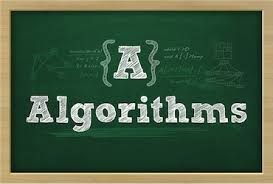21. Describe divide and conquer paradigm.

When a problem is solved using a divide and conquer algorithm, it is subdivided into one or more subproblems which are all similar to the original problem in such a way that each of the subproblems can be solved independently. In the end, the solutions to the subproblems are combined in order to obtain the solution to the original problem.

22. Describe on short an insertion sorting algorithm.

An algorithm that sorts by insertion takes the initial, unsorted sequence and computes a series of sorted sequences using the following rules:
a) the first sequence in the series is the empty sequence
b) given a sequence S(i) in the series, for 0<=i

23. Which are the advantages provided by insertion sort?

a) simple implementation
b) efficient for small data sets
c) adaptive – efficient for data sets that are already substantially sorted: the time complexity is O(n + d), where d is the number of inversions
d) more efficient in practice than most other simple quadratic, i.e. O(n2) algorithms such as selection sort or bubble sort; the best case (nearly sorted input) is O(n)
e) stable – does not change the relative order of elements with equal keys
f) in-place – only requires a constant amount O( 1) of additional memory space
g) online – can sort a list as it receives it

24. Shortly describe the quicksort algorithm.

In quicksort, the steps performed are the following:
a) pick an element, called a pivot, from the list
b) reorder the list so that all elements with values less than the pivot come before the pivot, while all elements with values greater than the pivot come after it (equal values can go either way)
c) recursively sort the sub-list of lesser elements and the sub-list of greater elements

25. What is the difference between selection and insertion sorting?

In insertion sorting elements are added to the sorted sequence in an arbitrary order. In selection sorting, the elements are added to the sorted sequence in order so they are always added at one end.

26. What is merge sorting?

Merging is the sorting algorithm which combines two or more sorted sequences into a single sorted sequence. It is a divide and conquer algorithm, an O(n log n) comparison-based sorting algorithm. Most implementations produce a stable sort, meaning that the implementation preserves the input order of equal elements in the sorted output.

27. Which are the main steps of a merge sorting algorithm?

Sorting by merging is a recursive, divide-and-conquer strategy. The basic steps to perform are the following:
a) divide the sequence into two sequences of length
b) recursively sort each of the two subsequences
c) merge the sorted subsequences to obtain the final result

28. Provide a short description of binary search algorithm.

Binary search algorithm always chooses the middle of the remaining search space, discarding one half or the other, again depending on the comparison between the key value found at the estimated position and the key value sought. The remaining search space is reduced to the part before or after the estimated position.

29. What is the linear search algorithm?

Linear search is a method for finding a particular value in a list which consists of checking every one of its elements, one at a time and in sequence, until the desired one is found. It is the simplest search algorithm, a special case of brute-force search. Its worst case cost is proportional to the number of elements in the list; and so is its expected cost, if all list elements are equally likely to be searched for. Therefore, if the list has more than a few elements, other methods (such as binary search or hashing) may be much more efficient.

30. What is best-first search algorithm?

It is a search algorithm that considers the estimated best partial solution next. This is typically implemented with priority queues.